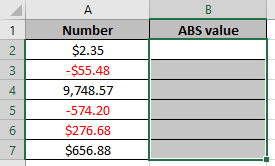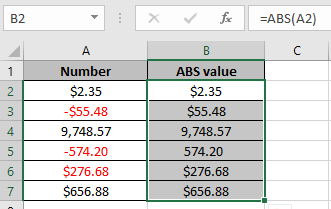# How to Convert negative number to positive number in Excel

In this article, we will learn about how to use absolute value function to convert negative numbers to positive numbers in Excel.

ABS function returns the Absolute value of a number, without its sign. Absolute value means negative number are converted to positive and the positive numbers stay unaffected.

Syntax:

=ABS(number)

Let’s run some tests on some numbers using ABS functionThe red cells are the negative numbers provided in different formatting styles.
Use the formula

=ABS(A2)Press Enter and Copy the formula in other cells using CTRL + D option.As you can see in the above snapshot, we got all the positive numbers using ABS function in Excel.

Hope you understood how to convert negative to positive numbers in Excel. Explore more articles on excel mathematical function here. Please state your queries in the comment box below.

Related Articles:

ABS - The Absolute Value Function

Popular Articles:

50 Excel Shortcuts to Increase Your Productivity

How to use the VLOOKUP Function in Excel

How to use the COUNTIF function in Excel

How to use the SUMIF Function in Excel

Terms and Conditions of use

The applications/code on this site are distributed as is and without warranties or liability. In no event shall the owner of the copyrights, or the authors of the applications/code be liable for any loss of profit, any problems or any damage resulting from the use or evaluation of the applications/code.